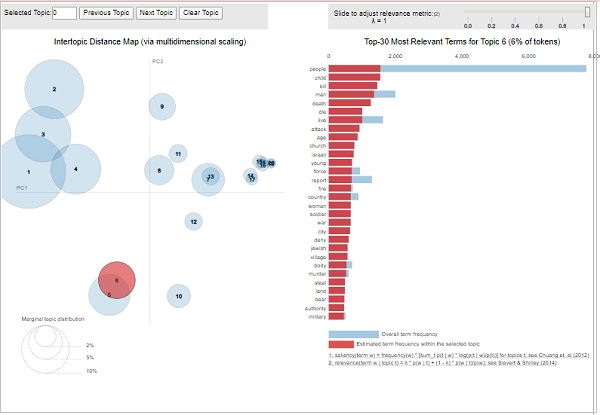# Gensim - Using LDA Topic Model

In this chapter, we will understand how to use Latent Dirichlet Allocation (LDA) topic model.

## Viewing Topics in LDA Model

The LDA model (lda_model) we have created above can be used to view the topics from the documents. It can be done with the help of following script −

```pprint(lda_model.print_topics())
doc_lda = lda_model[corpus]
```

## Output

```[
(0,
'0.036*"go" + 0.027*"get" + 0.021*"time" + 0.017*"back" + 0.015*"good" + '
'0.014*"much" + 0.014*"be" + 0.013*"car" + 0.013*"well" + 0.013*"year"'),
(1,
'0.078*"screen" + 0.067*"video" + 0.052*"character" + 0.046*"normal" + '
'0.045*"mouse" + 0.034*"manager" + 0.034*"disease" + 0.031*"processor" + '
'0.028*"excuse" + 0.028*"choice"'),
(2,
'0.776*"ax" + 0.079*"_" + 0.011*"boy" + 0.008*"ticket" + 0.006*"red" + '
'0.004*"conservative" + 0.004*"cult" + 0.004*"amazing" + 0.003*"runner" + '
'0.003*"roughly"'),
(3,
'0.086*"season" + 0.078*"fan" + 0.072*"reality" + 0.065*"trade" + '
'0.045*"concept" + 0.040*"pen" + 0.028*"blow" + 0.025*"improve" + '
'0.025*"cap" + 0.021*"penguin"'),
(4,
'0.027*"group" + 0.023*"issue" + 0.016*"case" + 0.016*"cause" + '
'0.014*"state" + 0.012*"whole" + 0.012*"support" + 0.011*"government" + '
'0.010*"year" + 0.010*"rate"'),
(5,
'0.133*"evidence" + 0.047*"believe" + 0.044*"religion" + 0.042*"belief" + '
'0.041*"sense" + 0.041*"discussion" + 0.034*"atheist" + 0.030*"conclusion" +
'
'0.029*"explain" + 0.029*"claim"'),
(6,
'0.083*"space" + 0.059*"science" + 0.031*"launch" + 0.030*"earth" + '
'0.026*"route" + 0.024*"orbit" + 0.024*"scientific" + 0.021*"mission" + '
'0.018*"plane" + 0.017*"satellite"'),
(7,
'0.065*"file" + 0.064*"program" + 0.048*"card" + 0.041*"window" + '
'0.038*"driver" + 0.037*"software" + 0.034*"run" + 0.029*"machine" + '
'0.029*"entry" + 0.028*"version"'),
(8,
'0.078*"publish" + 0.059*"mount" + 0.050*"turkish" + 0.043*"armenian" + '
'0.027*"western" + 0.026*"russian" + 0.025*"locate" + 0.024*"proceed" + '
'0.024*"electrical" + 0.022*"terrorism"'),
(9,
'0.023*"people" + 0.023*"child" + 0.021*"kill" + 0.020*"man" + 0.019*"death" '
'+ 0.015*"die" + 0.015*"live" + 0.014*"attack" + 0.013*"age" + '
'0.011*"church"'),
(10,
'0.092*"cpu" + 0.085*"black" + 0.071*"controller" + 0.039*"white" + '
'0.028*"water" + 0.027*"cold" + 0.025*"solid" + 0.024*"cool" + 0.024*"heat" '
'+ 0.023*"nuclear"'),
(11,
'0.071*"monitor" + 0.044*"box" + 0.042*"option" + 0.041*"generate" + '
'0.038*"vote" + 0.032*"battery" + 0.029*"wave" + 0.026*"tradition" + '
(12,
'0.048*"send" + 0.045*"mail" + 0.036*"list" + 0.033*"include" + '
'0.024*"book" + 0.024*"sell"'),
(13,
'0.515*"drive" + 0.052*"laboratory" + 0.042*"blind" + 0.020*"investment" + '
'0.011*"creature" + 0.010*"loop" + 0.005*"dialog" + 0.000*"slave" + '
'0.000*"jumper" + 0.000*"sector"'),
(14,
'0.153*"patient" + 0.066*"treatment" + 0.062*"printer" + 0.059*"doctor" + '

'0.036*"medical" + 0.031*"energy" + 0.029*"study" + 0.029*"probe" + '
'0.024*"mph" + 0.020*"physician"'),
(15,
'0.068*"law" + 0.055*"gun" + 0.039*"government" + 0.036*"right" + '
'0.029*"state" + 0.026*"drug" + 0.022*"crime" + 0.019*"person" + '
'0.019*"citizen" + 0.019*"weapon"'),
(16,
'0.107*"team" + 0.102*"game" + 0.078*"play" + 0.055*"win" + 0.052*"player" + '
'0.051*"year" + 0.030*"score" + 0.025*"goal" + 0.023*"wing" + 0.023*"run"'),
(17,
'0.031*"say" + 0.026*"think" + 0.022*"people" + 0.020*"make" + 0.017*"see" + '
'0.016*"know" + 0.013*"come" + 0.013*"even" + 0.013*"thing" + 0.013*"give"'),
(18,
'0.039*"system" + 0.034*"use" + 0.023*"key" + 0.016*"bit" + 0.016*"also" + '
'0.015*"information" + 0.014*"source" + 0.013*"chip" + 0.013*"available" + '
'0.010*"provide"'),
(19,
'0.085*"line" + 0.073*"write" + 0.053*"article" + 0.046*"organization" + '
'0.034*"host" + 0.023*"be" + 0.023*"know" + 0.017*"thank" + 0.016*"want" + '
'0.014*"help"')
]
```

## Computing Model Perplexity

The LDA model (lda_model) we have created above can be used to compute the model’s perplexity, i.e. how good the model is. The lower the score the better the model will be. It can be done with the help of following script −

```print('\nPerplexity: ', lda_model.log_perplexity(corpus))
```

### Output

```Perplexity: -12.338664984332151
```

## Computing Coherence Score

The LDA model (lda_model) we have created above can be used to compute the model’s coherence score i.e. the average /median of the pairwise word-similarity scores of the words in the topic. It can be done with the help of following script −

```coherence_model_lda = CoherenceModel(
model=lda_model, texts=data_lemmatized, dictionary=id2word, coherence='c_v'
)
coherence_lda = coherence_model_lda.get_coherence()
print('\nCoherence Score: ', coherence_lda)
```

### Output

```Coherence Score: 0.510264381411751
```

## Visualising the Topics-Keywords

The LDA model (lda_model) we have created above can be used to examine the produced topics and the associated keywords. It can be visualised by using pyLDAvispackage as follows −

```pyLDAvis.enable_notebook()
vis = pyLDAvis.gensim.prepare(lda_model, corpus, id2word)
vis
```

### OutputFrom the above output, the bubbles on the left-side represents a topic and larger the bubble, the more prevalent is that topic. The topic model will be good if the topic model has big, non-overlapping bubbles scattered throughout the chart.